favorite We need a little bit of your help to keep things running, click on this banner to learn more
Competitions

# Optimal Matrix Multiplication - 2

Given two matrices A and B, we can determine the matrix C = AB using the standard definition of matrix multiplication: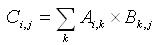The number of columns in the matrix A must be the same as the number of rows in the matrix B. Let matrix A has size m × n, matrix B has size n × p. Then matrix С will have the size m × p, and to calculate it you should perform m * n * p multiplications.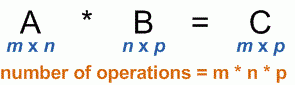For example, if size of A is 10 × 20, size of B is 20 × 15, it will take 10 × 20 × 15 = 3000 multiplications to compute matrix C.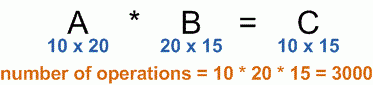Multiplication of more than two matrices can be done in multiple ways. For example, if X, Y and Z are matrices, then XYZ computation can be done either like (XY)Z or X(YZ). Let size of X be 5 × 10, size of Y be 10 × 20, and size of Z be 20 × 35.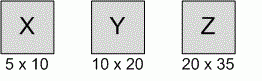Let's look at the number of multiplications required to compute the product using two different ways:

(XY)Z

• 5 × 20 × 10 = 1000 multiplications to determine the product (XY) that has size 5 × 20.
• Then 5 × 35 × 20 = 3500 multiplications to determine the final result.
• Total number of multiplications: 4500.

X(YZ)

• 10 × 35 × 20 = 7000 multiplications to determine the product (YZ) that has size 10 × 35.
• Then 5 × 35 × 10 multiplications to determine the final result.
• Total number of multiplications: 8750.

Clearly we'll be able to compute (XY)Z using fewer individual multiplications.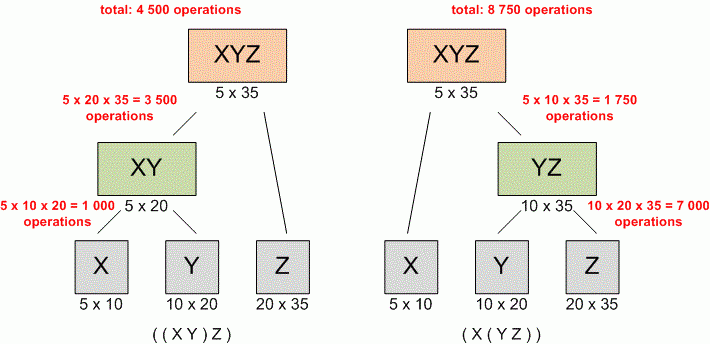Given the sequence of matrices to be multiplied, you are to determine the optimal order of their multiplication. Optimality in this problem is relative to the number of scalar multiplications required.

#### Input

Consists of multiple test cases. The first line of each test case contains the number n (n10) of matrices to be multiplied. It is followed by n pairs of integers, each pair giving the number of rows and columns in a matrix, matrix sizes are given in the order of their multiplication. The last test case contains n = 0 and should not be processed.

#### Output

Assume that matrices are named A1, A2,..., An. Your output for each input case must be a line containing a parenthesized expression clearly indicating the order in which the matrices should be multiplied. Prefix the output for each case with the case number (they are sequentially numbered, starting with 1). Your output must strongly resemble the samples shown below. If, by chance, there are multiple correct sequences, any of these will be accepted as a valid answer.

Time limit 1 second
Memory limit 128 MiB
Input example #1
3
1 5
5 20
20 1
3
5 10
10 20
20 35
6
30 35
35 15
15 5
5 10
10 20
20 25
0

Output example #1
Case 1: (A1 x (A2 x A3))
Case 2: ((A1 x A2) x A3)
Case 3: ((A1 x (A2 x A3)) x ((A4 x A5) x A6))